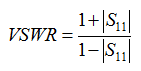RF - VSWR                                                  Home : www.sharetechnote.com VSWR stands for Voltage Standing Wave Ratio. To understand this term completely, you need to know what is 'Standing Wave'. You might have already learned about the standing wave in your high school physics class. Just refresh your mind, let me explain what the standing wave is.   Let's suppose that two waves with the same wavelength travels in opposite direction as shown below. One wave is represented as blue line and it is treveling in right direction. The other wave is represented as green line and it is treveling in left direction. As you learned in high school physics, these two waves would get summed up and the summed up wave is represented as red line. This summed wave changes over time only in terms of amplitude but does not change in phase. Simply put, the location of nodes where the amplitude is zero never changes. Since the location of nodes does not change, the red wave looks as if it stays at the same position meaning 'it STANDs there'. This is why the red wave is called 'Standing Wave'.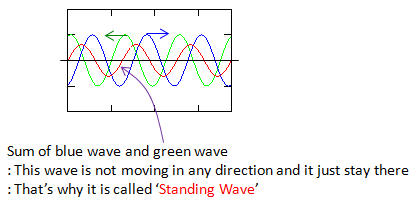With only single snapshot as shown above, it would be difficult for you to understand the standing wave intuitively. To understand the standing wave visually and to confirm if the description above, you would need multiple snapshots showing a sequence of transisions of the two traveling waves over time. You can draw these sequence by hand, but that would be very tedius... just using a simple matlab code as shown below, you can create a long sequence of snapshots showing how standing wave is created.   t = 0:pi/20:20*pi; ph = pi/20; r = 0.5;   for i=1:20 subplot(4,5,i); plot(t,sin(t+(i-1)*ph),'g-',t,r*sin(t-(i-1)*ph),'b-',t,sin(t+(i-1)*ph)+r*sin(t-(i-1)*ph),'r-'); axis([0 6*pi -2 2]); set(gca,'xticklabel',[]);set(gca,'yticklabel',[]); title(['(' int2str(i) ')']); end   One example sequence of snapshots of two traveling waves (blue one traveling right and green one traveling left. The red one is the wave formed by the summation of blue one and green one). First look at the red waves in all of the snapshots. Does the amplitude of the red wave changes in each of the snapshots ? --> Yes Does the frequency and wave length of the red wave changes  in each of the snapshots ? =-> No Does the location of nodes (the place where the value gets zero) changes  in each of the snapshots ? --> No   Let me give you some other questions to understand the characteristics of standing wave even further. What is the snapshot number showing the standing wave with largest amplitude ? --> It is (1). This is the snapshot where two traveling wave (blue and green) exactly overlap each other. What is the snapshot number showing the standing wave with smallest amplitude ? --> It is (11). This is the snapshot where two traveling wave (blue and green) alignes in such a way that the two waves has 180 degree phase difference. < Case 1 >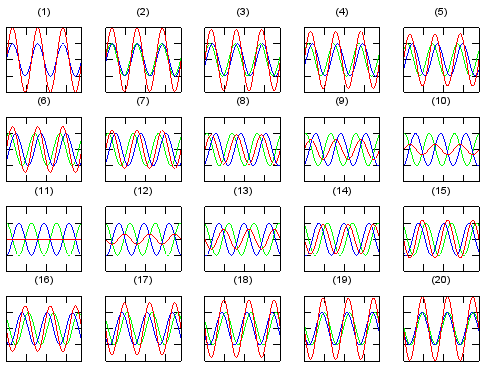In the example above, the amplitude of the two traveling wave are exactly same. What if the amplitude of the two traveling wave is different (wave length and frequency of the two waves are still same). Let me give the same question as I asked you above. Does the amplitude of the red wave changes in each of the snapshots ? --> Yes Does the frequency and wave length of the red wave changes  in each of the snapshots ? =-> No Does the location of nodes (the place where the value gets zero) changes  in each of the snapshots ? --> No   Let me give you some other questions to understand the characteristics of standing wave even further. What is the snapshot number showing the standing wave with largest amplitude ? --> It is (1). This is the snapshot where two traveling wave (blue and green) has the same phase. What is the snapshot number showing the standing wave with smallest amplitude ? --> It is (11). This is the snapshot where two traveling wave (blue and green) alignes in such a way that the two waves has 180 degree phase difference. But unlike < Case 1>, the amplitude of the standing wave does not become zero in this case because the amplitude of the two traveling wave is different.   < Case 2 >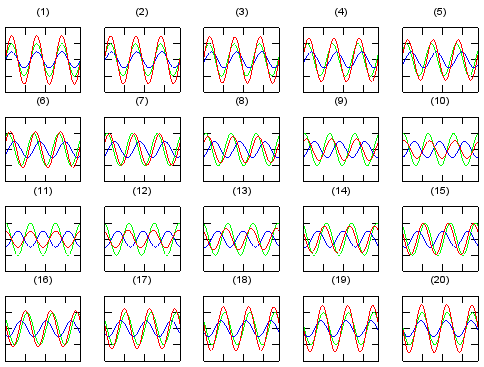Now let's take the ratio of the standing wave amplitude for two snapshots with the smallest amplitude and the largest amplitude. In case of the two examples we saw above, it is the ratio of standing wave amplitude for snapshot (1) and (11). This ratio of standing wave with maximum amplitude and minimum amplitude is called 'Standing Wave Ratio'. Standing Wave Ratio for the first and second example that we saw above can be illustrated as below. (The upper one represents the min/max standing wave amplitude difference for < Case 1 > and the lower one represents the min/max standing wave amplitude difference for < Case 2 > )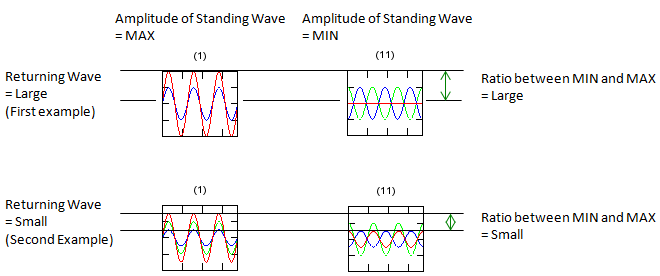If the two traveling wave represents the voltage of a signal, the Standing Wave Ratio is called 'Voltage Standing Wave Ratio (VSWR)'. As you see in the two examples, you may notice the closer amplitude of the traveling wave are, the larger SWR. In other words, if you have larger SWR, the amplitude of the two traveling wave is similar. If you have smaller SWR, it means the amplitude of the traveling waves are much different (one is big and the other is very small).   Where we see these standing wave most commonly ? The most common situation is the case where a traveling wave hits the boundary of another medium. In such a case, some portions of wave would transfer to the other medium but some portion of the wave would get reflected back as illustrated below.This reflected wave would travel in opposite direction to the original wave but it's wave length (frequency) remain same as the original wave. As a result, the original wave and the reflected wave form a standing wave.   Based on how much portion of the original wave gets reflected from the boundary (this is described by Reflection Coefficient), you can calculate the VSWR using the following equations.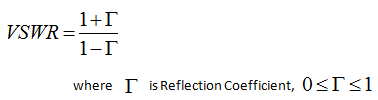What is the intuitive relationship between the reflection coefficient and VSWR ? You can guess from the following examples. In short, if you have larger reflection coefficient, you would get larger VSWR and if you have smaller reflection coefficient, you would get smaller VSWR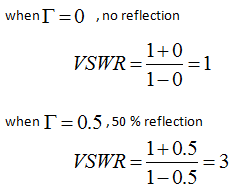Since reflection coefficient is tighly related to S11, you can represent VSWR using S11 as well, as shown below.